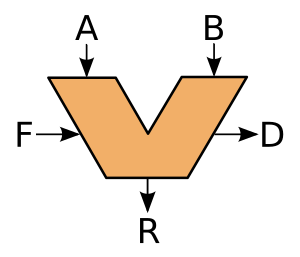# Arithmetic logic unit facts for kids

Kids Encyclopedia Facts
(Redirected from ALU)A typical schematic symbol for an ALU: A & B are the data (registers); R is the output; F is the Operand (instruction) from the Control Unit; D is an output status

In computing, an arithmetic logic unit (ALU) is a digital circuit that performs arithmetic and logical operations. The ALU is a basic building block of the central processing unit (CPU) of a computer, even the simplest microcontrollers contain one for purposes such as timing.

Now, modern microprocessors, microcontrollers and CPUs have very powerful and very complex ALUs. Also, more recent CPUs may contain up to more than 4 ALUs (see superscalar CPUs).

Mathematician John von Neumann proposed the ALU concept in 1945.

## Images for kidsArithmetic logic unit Facts for Kids. Kiddle Encyclopedia.